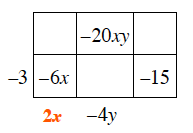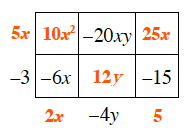### Home > CCAA8 > Chapter 6 Unit 7 > Lesson CCA: 6.2.1 > Problem6-59

6-59.

Copy each generic rectangle below and fill in the missing parts. Then write an equation showing the area as a product and the area as a sum.

1.  Generic rectangle left edge top blank interior top left blank interior top middle$-20xy$ interior top right blank left edge bottom$-3$ interior bottom left $-6x$ interior bottom middle blank interior bottom right $-15$ bottom edge left blank bottom edge middle$-4y$ bottom edge right blank
• What should you multiply $−3$ by to get $−6x$?
Use the same logic to fill in the sides of the rectangle. A generic rectangle with two rows of 3. The left edge bottom is negative 3. The bottom edge far left is 2, X. The bottom edge middle is negative 4, Y. The interior is labeled as follows: Upper row middle is negative 20 x, y. The lower row far left is negative 6, x. The lower row far right is negative 15.

• Fill in the remaining empty squares in the rectangle. Added to the generic rectangle: The left edge top is negative 5, x. The bottom edge far right is 5. The interior is labeled as follows: Upper row far left is negative 10 x, squared. The upper row far right is 25, X. The lower row middle is 12, y.

• To write the equation as a product, multiply the polynomials on the outside of the rectangle.

• To write the equation as a sum, add the values inside the rectangle.$\left(5x − 3\right)\left(2x − 4y + 5\right) = 10x^{2} − 20xy + 19x +12y − 15$

1.  Generic rectangle left edge top$x$ interior top left $x^2$ interior top right blank left edge bottom blank interior bottom left blank interior bottom right $12$
• There is more than one possible solution.